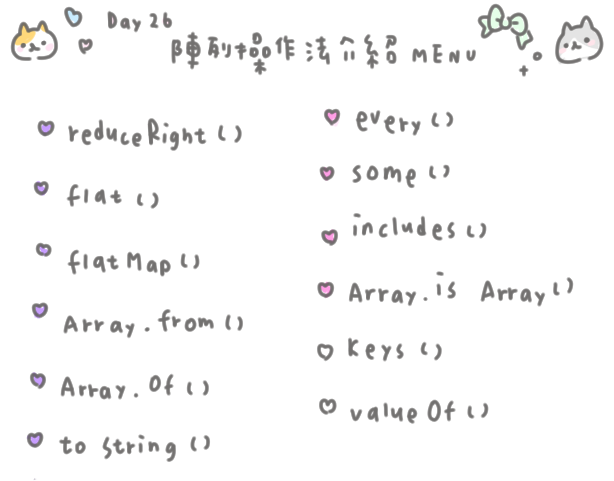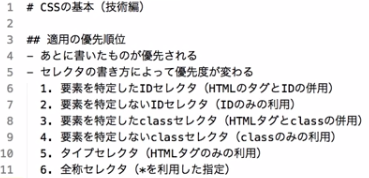DAY 26
0
Modern Web

二十六日目：JavaScript　陣列操作　終ノ章　 ⑅( ◜ᴗ◝)❤︎

O HA YOoooo (U 'ᴗ' U)⑅　SONYKO 打油⑅• 紫色 ♡ 是 會回傳新陣列 or 新的値 的操作法。
• 粉紅 ♡ 是 會判斷並回傳布林値的操作法。
• 白色 ♡ 是其它。

⑅　reduceRight ()

reduceRight 會對陣列中每個元素，由右至左傳入指定的函數，最後返回累加後的値。

// 你ㄉ陣列
let arr = ['あ','い','う','え','お']
let new_arr = arr.reduceRight(function(a,b){
return a + b
})
console.log(new_arr)    // "おえういあ"
• 昨天也有說到的、函式內的參數 a 你可以當他是 new_arr 、new_arr 去累加 b 。
• console 結果可以看出它是由右至左執行。

let arr = ['あ','い','う','え','お']
let new_arr = arr.reduce(function(a,b){
return a + b
})
console.log(new_arr)    // "あいうえお"

let num = [1,2,3,4,5]
let total = num.reduceRight((a,b)=>{ return a+10 },0)

console.log(total)  // 15

⑅　flat ()

flat 是用來將 巢狀陣列 攤平用的操作法。

let arr = [1,2,[3,4]]
let new_arr = arr.flat( 攤開層數 )

// 巢爆
let arr3 = [1,2,[3,4,[5,6,[7,8,9]]]]

// 先來攤開一層看看會變怎樣
let new_arr = arr3.flat(1)
console.log(new_arr) // [1,2,3,4,[5,6,[7,8,9]]]

// 攤開兩層的話
let new_arr2 = arr3.flar(2)
console.log(new_arr2) // [1,2,3,4,5,6,[7,8,9]]

// 如果不指定層數的話呢
let arr_f = arr3.flat()
console.log(arr_f) // [1,2,3,4,[5,6,[7,8,9]]]

⑅　如果沒有指定攤開層數、就只會攤開一層。

⑅　flatMap ()

flatMap 就是 Map 加上 flat (ˉ ˘ ˉ; )

⑅　來看 map + flat ：

/* 範例陣列 */
let friend_oshi = [
{ name:'小明',lover:['泉','真']},
{ name:'小美',lover:['零','凛月']},
{ name:'俊偉',lover:['英智','藍良']},
{ name:'sonyko',lover:['嵐','泉']}
]

/* map */
let oshi =  friend_oshi.map(i => i.lover)
console.log(oshi)
// [['泉','真'],['零','凛月'],['英智','藍良'],['嵐','泉']]

/* flat */
let oshi_f = oshi.flat()
console.log(oshi_f)
// ['泉','真','零','凛月','英智','藍良','嵐','泉']

⑅　來看 flatMap ：

/* 同上方陣列 */
let oshi_fm = friend_oshi.flatMap(i =>i.lover)
console.log(oshi_fm)
// ['泉','真','零','凛月','英智','藍良','嵐','泉']

⑅　Array.from ()

let a = '12345'
let b = Array.from(a)
console.log(b)  // ['1','2','3','4','5']

let b = Array.from(a,function(i){
return i+`隻喵`
})
console.log(b)
// ['1隻喵','2隻喵','3隻喵','4隻喵','5隻喵']

⑅　Array.of ()

Array.of 可以將小括號內容通通變陣列 。

let a = 9527
let arr = Array.of('哇',a,'噴火龍',100,'等')
console.log(arr)
// ['哇',9527,'噴火龍',100,'等']

⑅　to String ()

let arr = ['a', 'b', 'c' ,'d']
let str = arr.toString()
console.log(arr)   // " a,b,c,d "

⑅　every ()

every 會將陣列的每一個元素帶入函式做判斷。

// false 的例子
let num = [9,5,2,7]
function sum(i){
return i > 3
}
console.log(num.every(sum))  // false

// true 的例子
let num2 = [9,5,22,7]
function sum(i){
return i > 3
}
console.log(num2.every(sum))  // true

⑅　some ()

let num = [9,5,2,7]
function sum(i){
return i > 3
}
console.log(num.some(sum))  // true
// 即使 2 < 3 也是 true

⑅　includes ()

includes 有兩個參數：一是輸入要檢查的値、二是從哪裡開始檢查。

let num = [5,4,3,8,8,7]
console.log (num.includes(2))  // false
console.log (num.includes(3))  // true
console.log (num.includes(5,2))  // false 陣列第2筆之後沒有5

⑅　Array.isArray ()

let a = [1,2,3]
let b = '小否龍'
let c = 10
let d = { name:'乂煞氣a誠乂', lv: 175 }

console.log(Array.isArray(a))  // true
console.log(Array.isArray(b))  // false
console.log(Array.isArray(c))  // false
console.log(Array.isArray(d))  // false

⑅　keys ()

let a = ['A', 'B', 'C', 'D', 'E']
let b = a.keys()

for (let key of b ){
console.log (key)
}
/*
0
1
2
3
4
*/

⑅　valueOf ()

let num = [1,2,3]
let vO_num = num.valueOf()
console.log(vO_num)  // [1,2,3] 尚未更動到

num.reverse()
console.log(vO_num) // [3,2,1] 表示更動到原陣列ㄌ

୨୧(U 'ᴗ' U) SONYKO的　前端日文小教室 ♡

⑅　音檔復活 °♡

「 師傅、你昨天騙我！畫面右上角根本沒東西。」

「 怎麼可能？ 不然你找找看左上角吧 」

ε≡≡ﾍ( ´Д`)ﾉ♫　第 3 行開始：

• 適用の優先順位
適用 就是 採用、被使用 的意思。 這邊就是我們中文講的 權重
順位 就是 順序的意思 。

• あとに書いたものが優先される
寫在下方的扣會優先採用 。
也就是我們CSS 後面的程式碼會蓋掉前面的 (權重相同的情況下)

• セレクタの書き方によって優先度が変わる
優先度會依選擇器的寫法改變
セレクタ（ selector ）選擇器

• 要素を特定したIDセレクタ ( HTMLのタグとIDの併用 )
指定元素的 ID 選擇器 （ HTML 標籤與 ID並用 ）
就是像 p#text 這樣的寫法 ( 我自己是沒用過 )

• 要素を特定しないIDセレクタ( IDのみの利用 )
不指定元素的ID 選擇器 ( 只使用 ID )

《 接下來都是一樣的文法、所以就略過哩 (U 'ᴗ' U)♡》

• タイプセレクタ ( HTMLタグのみの利用 )
Type Selector 元素選擇器、型態選擇器 （ 只使用 HTML 標籤 ）

• 全称セレクタ（ * を利用した指定 ）
通用選擇器 （ 使用 * 去指定 ）